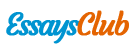# Ktratc

Autor:   •  October 26, 2018  •  1,266 Words (6 Pages)  •  103 Views

Page 1 of 6

...

Through calculation, the obtained percentage error of the mass and the angle of equilibrant were 3.60% and 8.7% respectively.

Case 4

Setting the two added hanging mass to be perpendicular with each other and adjusting their magnitude at the same time, the ring was kept at the center. The obtained analytical value for the mass and the angle of the equilibrant was 188.19g and 199.95° respectively. This implies that in this case, the ring will be more centered if the mass and the angle of the equilibrant is 188.19g and 199.95° respectively.

Through calculation, the obtained percentage error of the mass was 3.60% and the percentage error of the angle was 8.7%.

Case 5

Using three different pulleys with varying magnitudes and positions, it was possible to KTRATC. The values of the mass and the angle of the three hanging masses are as follows: 234.62g at an angle of 251°, 528.42g at an angle of 25°, and 249.01g at an angle of 155°. Using these values, the calculated analytical value of the equilibrant was 178.55g and 199.95° for the angle.

Through calculation, the obtained percentage error of the mass and the angle of the equilibrant were 12.03% and 8.7%.

In all the different cases, the errors can be accounted for several factors. First, the force table might not have been balanced. The force table was being accidentally bumped by the students because of the lack in space in the laboratory. Second is the friction on the pulleys. Third is the parallax error. The indication of the position of the string might not correspond at the right line of the angle measurement. Fourth is the misaligned central rod of the force table. This had caused many of the parallax errors. Fifth is the adjustment of the pulleys. The pulleys are adjusted at very small degrees in order to get the best possible angular position. Sixth is the friction on the ring. The friction might have caused the strings to not pass at a common point at the ring center. And lastly, the mass of the strings were ignored.

Using two hanging masses with equal magnitude, the unknown mass of a block was identified. On the first trial, the calculated mass of the block was 292.05g. On the second trial, the calculated mass of the block was 418.84g. The actual mass of the block was 308.05g. The first trial provided 5.29% error and the second trial provided 36% error in the calculated mass of the block.

Conclusion

It is possible to KTRATC in the different cases provided – different magnitudes of hanging mass and positions or angles even though there were some sources of errors present during the experiment. These were evidently seen wherein the computed percentage errors were only minimal.

To KTRATC, the magnitude (force) should be equal to zero wherein the resultant vector must be equal to that of the equilibrant. This is reflected on positioning each hanging mass. At some point, the ring was positioned further on one side so the mass must be increased on the side opposite to the favored one. Thus, magnitude or position alone would not suffice to achieve the aim to KTRATC. Both variables are dependent in analyzing vectors.

...